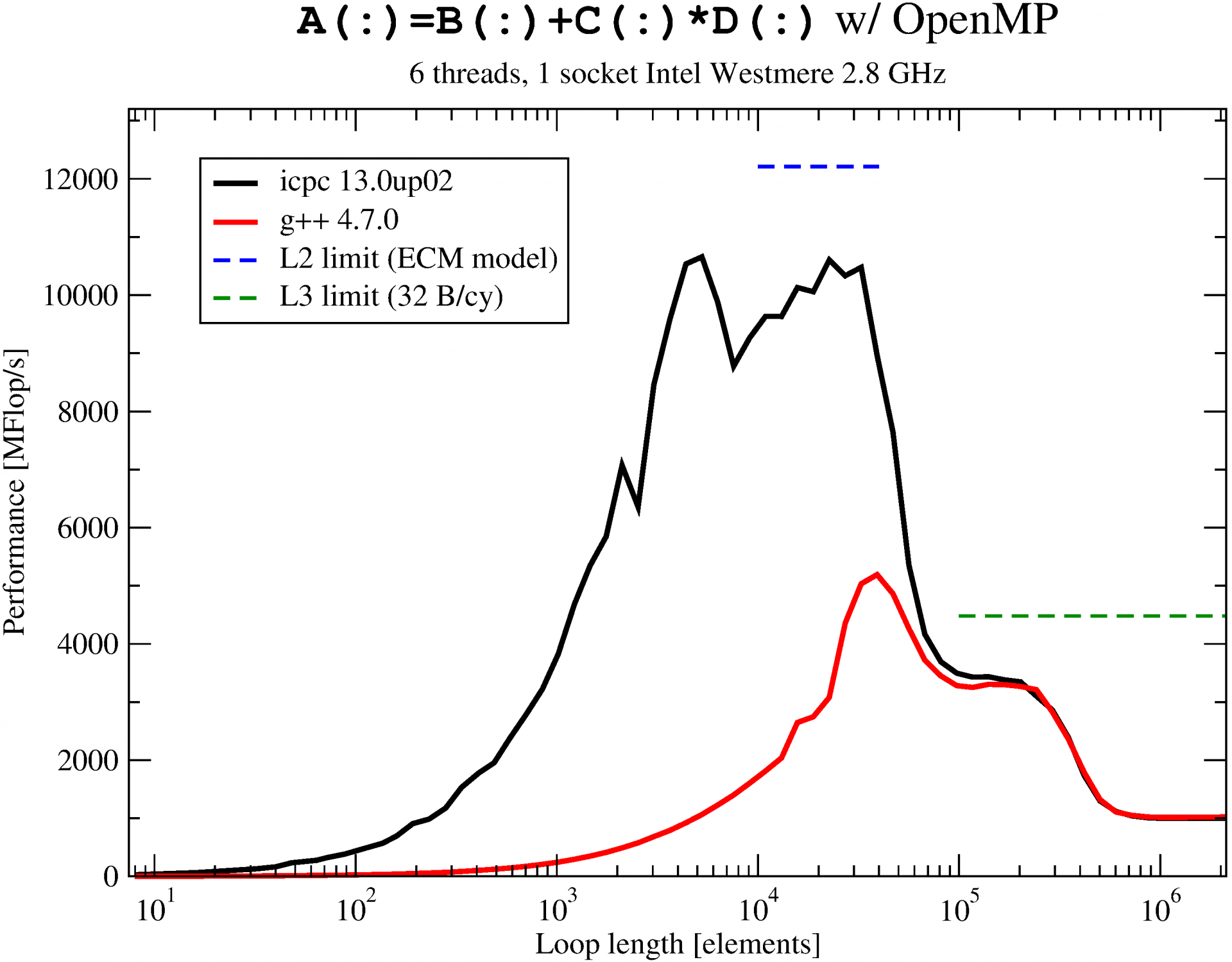21st September 2013

# Georg Hager's Blog: Intel vs. GCC for the OpenMP vector triad: Barrier shootout!

Georg Hager's Blog posted an illustrative article on icc versus g++ performance w.r.t. OpenMP. Dr. Georg Hager is one of the authors of Introduction to High Performance Computing for Scientists and Engineers.

Measurement of

``````double precision, dimension(N) :: a,b,c,d
! initialization etc. omitted
s = walltime()
!\$omp parallel private(R,i)
do R=1,NITER
!\$omp do
do i=1,N
a(i) = b(i) + c(i) * d(i)
enddo
!\$omp end do
enddo
!\$omp end parallel
e=walltime()
MFlops = R*N/(e-s)/1.e6
``````

gives